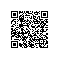# 用c语言手搓一个500+行的类c语言解释器: 给编程初学者的编译器教程（1）- 目标和前言

https://github.com/yunwei37/tryC

## 一个小目标

• 选择和循环的流程控制语句
• 支持的数据类型：双精度浮点数、字符型、字符串、浮点数数组
• 支持函数和变量的定义、函数的递归调用、嵌套作用域

（如果看不懂下面这段也没关系，可以略过啦）

### 解释器可运行的代码示例

# Fibonacci sequence
func fun{
if(x <= 2){
return(1);
}
y = 0;
x = x - 1;
y = fun(x);
x = x - 1;
return(y + fun(x));
};

# save the Fibonacci sequence of 1 to 15 in an array
array arr(15);
x = 1;
while( x <= 15 ){
arr[x - 1] = fun(x);
x = x + 1;
}

puts("Fibonacci sequence:");
# print the Fibonacci sequence of 1 to 15
i = 0;
while(i < 15){
print(arr[i]);
i=i+1;
}


（起名困难x）这个小玩意我们就随便叫它tryC吧，当做是一个小的尝试。

### 部分语言规则：

• 注释在一行内，以‘#’开头；
• 语句以‘;’结尾
• 赋值语句类型：

x = 123.4;
x = 'c';
x = "hello world!";
• 循环语句：

while( bool ){
statements
}
• 选择语句：

if( bool ){
statements
}

if( bool ){
statements
}else{
statements
}
• 定义函数：函数参数在定义中不出现，在调用中获取；返回值为double

func function_name{
...
return(expression);
}

• 定义数组：

array array_name(array_length);

• 输入输出：

puts(string);
print(num);
read(num);

## 写在前面

### 需要了解的前置知识

• c语言的指针、函数指针、结构体等
• 递归的思想

### 心理准备

• 写一个600行的解释器虽然不算什么大工程，但相关的原理还是稍微有些复杂的，可能需要多花一些时间理解程序的运行过程；
• 代码可能难以调试，尤其在没有生成中间代码的情况下；

### 参考资料

• 《编译原理及其实践》
• c4

用四个函数和很少的代码就完成了功能相当完善的 C 语言编译器, 并且能够自举；我自己写作的时候也借鉴了c4的许多实现思想；

• 手把手教你构建 C 语言编译器

对c4的一个重写版，附有详细的中文教程

• Let's Build a Compiler, by Jack Crenshaw

一个英文的初学者教程，讲解如何实现一个编译器使用钉钉扫一扫加入圈子
+ 订阅